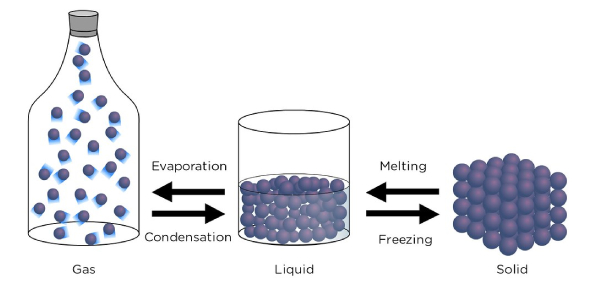# Nature Of Matter Unit Test

40 Questions | Total Attempts: 2010SettingsNature of Matter

• 1.
Which of the following is a characteristic property of an element?
• A.

Boiling point

• B.

Volume

• C.

Shape

• D.

Weight

• 2.
A cubic centimeter is a unit for measuring
• A.

Length

• B.

Mass

• C.

Volume

• D.

Density

• 3.
Which of the following is NOT a characteristic of a compound?
• A.

Has different properties from the elements that formed it

• B.

Is a pure substance

• C.

Different samples have different properties

• D.

Can be represented by a formula

• 4.
An unchanging measurement of the amount of matter an object contains is its
• A.

Mass

• B.

Volume

• C.

Density

• D.

Length

• 5.
Characteristic properties for a substance
• A.

Depends on volume

• B.

Never change

• C.

Depends on temperature

• D.

Differ on different planets

• 6.
A (n) _____ is a well-blended mixture that appears to be a single substance.
• A.

Compound

• B.

Pure Substance

• C.

Element

• D.

Solution

• 7.
A chemical bond is
• A.

A group of atoms that are joined together

• B.

The basic particle of matter

• C.

The force that holds two atoms together

• D.

A substance formed from the chemical combination of two or more atoms

• 8.
Which of the following is an example of a chemical change?
• A.

Melting butter

• B.

Breaking Glass

• C.

Mixing milk and chocolate syrup

• D.

Burning Leaves

• 9.
Which of the following is NOT true of atoms?
• A.

They are a compound of molecules

• B.

They make up elements

• C.

They combine to form compounds

• D.

They are extremely small

• 10.
The measure of the average energy of motion of the particles in a substance is
• A.

Temperature

• B.

Pressure

• C.

Chemical Energy

• D.

Viscosity

• 11.
A liquid has a
• A.

Constant temperature

• B.

Crystal structure

• C.

Definite shape

• D.

Definite volume

• 12.
The change from a gas to a liquid is called
• A.

Evaporation

• B.

Melting

• C.

Condensation

• D.

Sublimation

• 13.
Which of the following is NOT a characteristic of crystalline solids?
• A.

Particles arranged in repeating patterns

• B.

Becomes softer and softer as temperature rises

• C.

• D.

Has a distinct melting point

• 14.
Boyle’s Law state that as the pressure of gas increases, its
• A.

Volume increases

• B.

Volume decreases

• C.

Temperature increases

• D.

Temperature decreases

• 15.
Which of the following is NOT an example of vaporization?
• A.

Dry air gains water as it moves over the ocean

• B.

A bubble forms as water boils

• C.

Wet pavement dries after a rain shower

• D.

Water droplets form on a mirror

• 16.
A substance which takes the shape of volume of its container is a
• A.

Solid

• B.

Gas

• C.

Liquid

• D.

Crystal

• 17.
Which of the following is an example of a chemical change?
• A.

Twisting a wire

• B.

Water freezing

• C.

A pond drying up

• D.

Burning gasoline producing carbon dioxide

• 18.
As the gas in a rigid container is heated, its
• A.

Pressure increases

• B.

Volume increases

• C.

Pressure decreases

• D.

Volume decreases

• 19.
A liquid with a low viscosity
• A.

Has a definite shape

• B.

Flows quickly

• C.

Flows slowly

• D.

Fills it's container

• 20.
An element’s properties can be predicted from its
• A.

Number of protons

• B.

Number of neutrons

• C.

Atomic mass

• D.

Location in the periodic table

• 21.
In the ___ state of matter, electrons are stripped away from the nuclei.
• A.

Gas

• B.

Plasma

• C.

Liquid

• D.

Solid

• 22.
If a substance is ____, it can be pulled or drawn into a wire.
• A.

Ductile

• B.

Malleable

• C.

Liquid

• D.

Solid

• 23.
The sun and many other stars are made mostly of the element
• A.

Carbon

• B.

Iron

• C.

Hydrogen

• D.

Oxygen

• 24.
A (n) _____ is a positively charged particle in an atom’s nucleus.
• A.

Electron

• B.

Neutron

• C.

Plasma

• D.

Proton

• 25.
Which o f the following is NOT a characteristic of most metals?
• A.

Brittle

• B.

Good conductor

• C.

Ductile

• D.

Malleable

Related TopicsBack to top